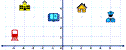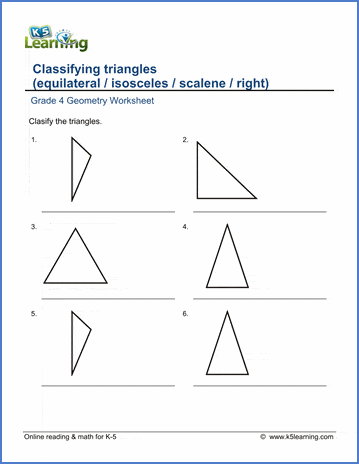# 4th Grade Math Worksheets: Geometry

## Free geometry worksheets

Our grade 4 geometry worksheets cover topics such as classifying angles, triangles and quadrilaterals, areas and perimeters and coordinate grids.

## Classifying angles, triangles & quadrilaterals

Classify angles
Acute, obtuse or right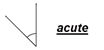Classify triangles by their sides
Equilateral, isosceles, or scalene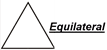Squares, rectangles, rhombuses, or parallelograms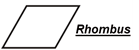## Area & perimeter

Perimeter and area using a grid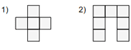Perimeter and area of rectangles - given two sides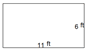Perimeter and area of irregular rectangular shapes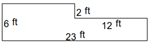## Circles

Circumference of circles - given the radius or diameter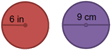## Symmetrical shapes

Lines of symmetry - draw lines of symmetry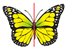Reflections - Identify & draw reflections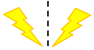Tessellations - which shapes form tessellations?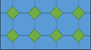Rotational symmetry - recognize rotational symmetry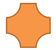## 3D Shapes

Volume of rectangular prisms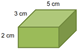## Coordinate grids

Plot points on a coordinate grid  - 1st quadrant only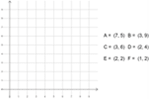Plot points on a coordinate grid - 4 quadrants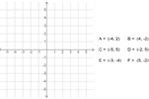Mystery pictures on a coordinate grid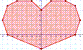Mystery messages on a coordinate grid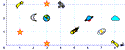Read maps on a coordinate grid